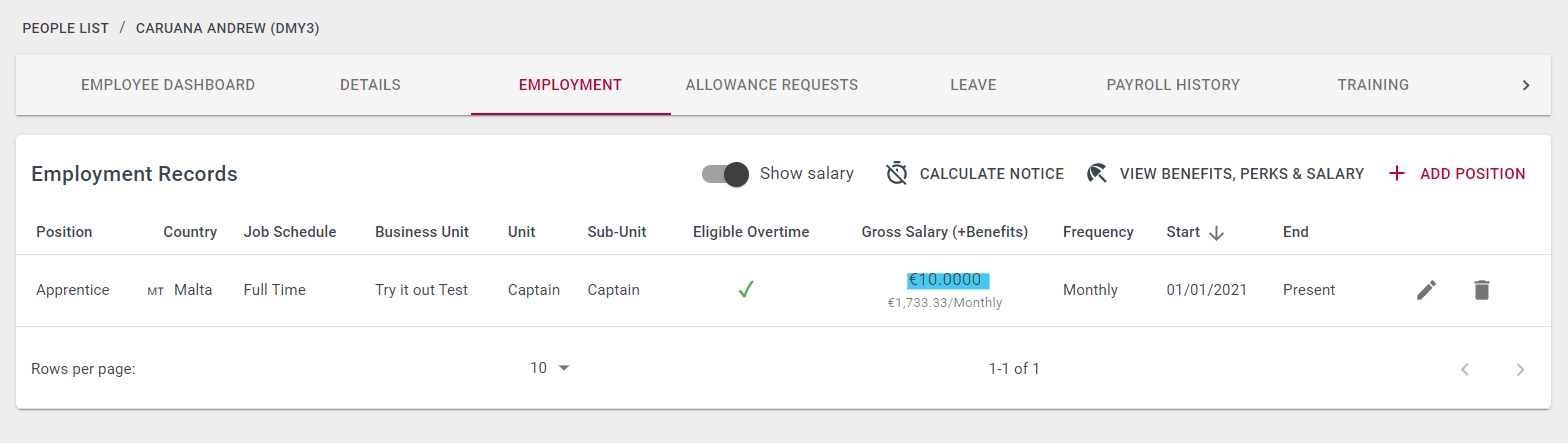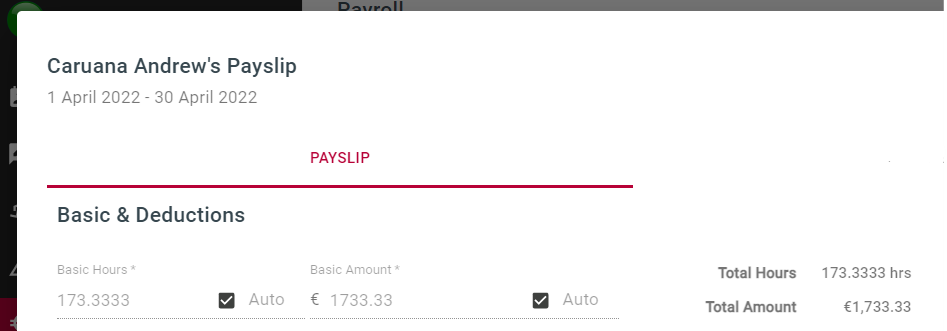# How is the Basic Amount calculated?Talexio Support
• Updated

## Overview

The Basic Amount is the amount owed to the employee which is directly related to the hours worked. This does not include leave hours/leave amount.

## Calculation

The basic amount is calculated as Basic Hours * Hourly rate

For example:

Andrew earns €10/hour.His basic hours worked in April are 173.3333. Therefore, €10 * 173.3333 = €1733.33.## Exceptions

• If there is more than one position history for the employee for the period covered by the payslip, the Basic Amount is shown as an average of the different hourly rates within that month. Ex. 50% of the month earning €10/hr and 50% of the month with earning €11/hr - the basic amount will show an average hourly rate of €10.5/hr for that month.
• If there is more than one position history for the employee for the period covered by the payslip, each having a different work schedule, the same principle as the above applies. Please note that in this case, the Basic Hours may also not add up to 173.3333 (for a 40hr/week, 5 days/week).
• If the employee worked less hours than required, or booked more leave hours than having hours worked, within that month, then the Basic amount will show as zero.
• If the employee did not work the full month, Basic Amount is calculated as follows:

(Salary/full-time hours based on 12 months) * Hours worked that month

Ex:  (€20800/2080 hours) * 64 hours worked = €640

Share:
<% if (previousArticle || nextArticle) { %>
<% if (previousArticle) { %>
<% if (previousTitle) { %>

#### <%= previousTitle %>

<% } %> <%= previousArticle.title %>
<% } %> <% if (nextArticle) { %>
<% if (nextTitle) { %>

#### <%= nextTitle %>

<% } %> <%= nextArticle.title %>
<% } %>
<% } %>
<% if (allItems.length > 1) { %>